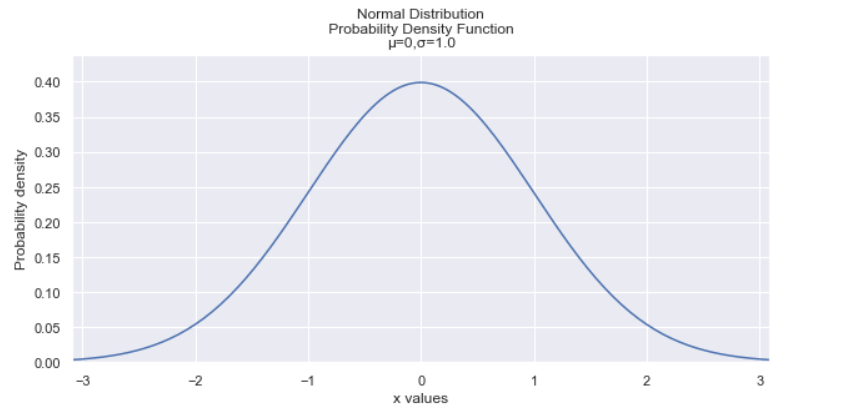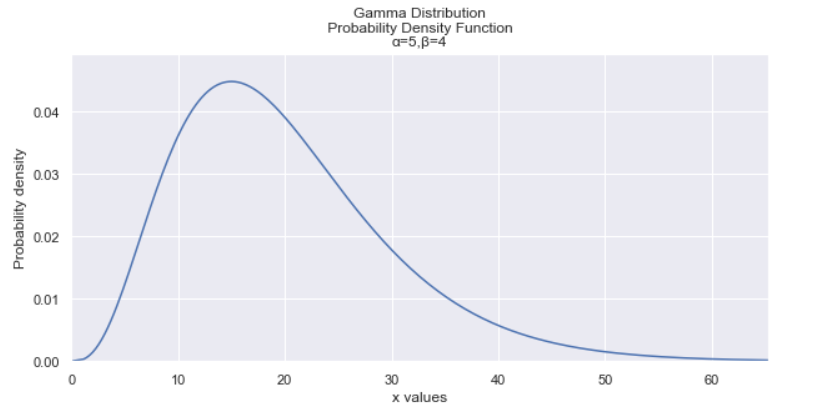Image Created by Author.

Probability distributions were so daunting when I first saw them, partly because there are so many of them, and they all have such unfamiliar names.

Fast forward to today, and I realized that they’re actually very simple concepts to understand when you strip away all of the math behind them, and that’s exactly what we’re going to do today.

Rather than getting into the mathematical side of things, I’m going to conceptually go over what I believe are the most fundamental and essential probability distributions.

By the end of this article, you’ll not only learn about several probability distributions, but you’ll also realize how closely related many of these are to each other!

First, you need to know a couple of terms:

• probability distribution simply shows the probabilities of getting different outcomes. For example, the distribution of flipping heads or tails is 0.5 and 0.5, respectively.
• discrete distribution is a distribution in which the values that the data can take on are countable.
• continuous distribution, on the other hand, is a distribution in which the values that the data can take on are not countable.

### 1. Normal DistributionImage created by Author.

The normal distribution is arguably the most important distribution to know because many phenomena fit this distribution. IQs, heights of people, shoe size, birth weight are all examples that have a normal distribution.

The normal distribution has a bell-shaped curve and has the following properties:

• It has a symmetric bell shape.
• The mean and median are equal and are both located at the center of the distribution.
• ≈68% of the data falls within 1 standard deviation of the mean, ≈95% of the data falls within 2 standard deviations of the mean, and ≈99.7% of the data falls within 3 standard deviations of the mean.

The normal distribution is also an integral part of statistics, as it is the basis of several statistical inference techniques, including linear regression, confidence intervals, and hypothesis testing.

### 2. T-distribution

The t-distribution is similar to the normal distribution but is generally shorter and has fatter tails. It is used instead of the normal distribution when the sample sizes are small.

One thing to note is that as the sample size increases, the t-distribution converges to the normal distribution.

### 3. Gamma DistributionImage…